# Cost Geometry 造價幾何

Go to End

-> Top

## 1. Note

• 27/2/2023: Revised.
• 20/2/2023: Split from "Estimating and Pricing".
-> Top

## 2. Cost vs quantity

### Not a straight-line relationship between costs and quantities

1. Cost = f(weight) + f(volume) + f(area) + f(length) + f(number) + f(quality) + f(quality) + f(time) + f(others).
2. But, the pricing unit of an item of work or material is based on one kind of unit of measurement.
3. This means that all other costs not related to that unit have to be converted to use that unit as the base.
4. These other cost factors do not change in direct proportion to the pricing unit.

### ​​​​​​​Total cost vs quantity

1. The total cost of factory production (and therefore sales of materials and goods) does not increase in proportion to the increase in the quantity produced, since there are some initial fixed costs (e.g. factory, equipment, rental deposits) and recurring fixed costs (e.g. monthly rents, additional equipment when the existing capacity is exceeded) not in proportion to the quantity to be included.
2. The total sales value may also not increase in proportion to the increase in the quantity sold (e.g. bulk discount, delivery vehicle not used to full capacity).
3. The total cost is usually higher than the total sales value initially, but the total sales value must rise up to exceed the total cost eventually for the business to be viable.

### Linear items

1. For linear items, there can be fixed costs which do not change when the length changes.
2. The unit rate per length can decrease if the length is increased without any change to the fixed components.
• e.g. 10 m @ \$4/m + 2 No. @ \$5/No. = \$40 + \$10 = \$50;
• \$50 / 10 m = \$5.00/m;
• while 15 m @ \$4/m + 2 No. @ \$5/No. = \$60 + \$10 = \$70;
• \$70 / 15 m = \$4.67/m.

(revised, 18/2/2023)

### Superficial items

1. For superficial items, the perimeter length increases less rapidly than the increase in the superficial area (300% vs 800% in the table below):
•2. The ratio of perimeter length / area decreases if the area is increased.
3. This means the unit rate per superficial area would have the same effect.
4. However, for the same area, the perimeter length can increase considerably if the shape is elongated:​​​​​​​​​​​​​​
•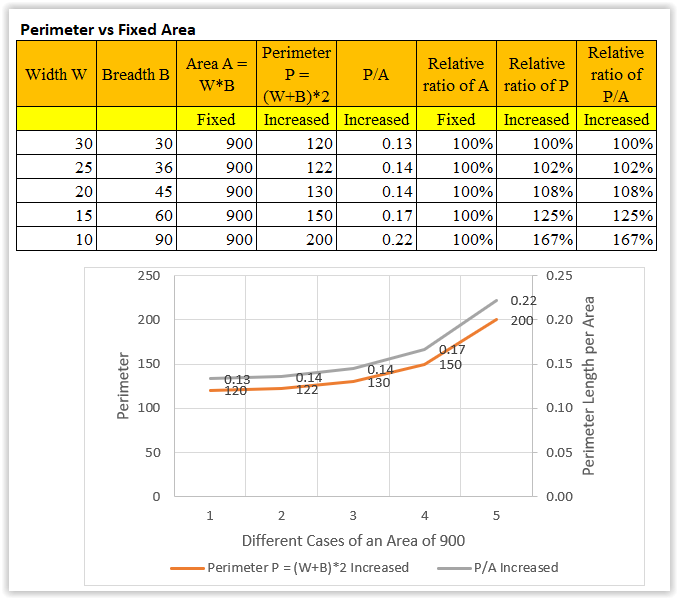5. The shape is important.

### Cubic items

1. For cubic items, the rates of change of the side area and volume relative to the base area would depend on which dimension is changed:
•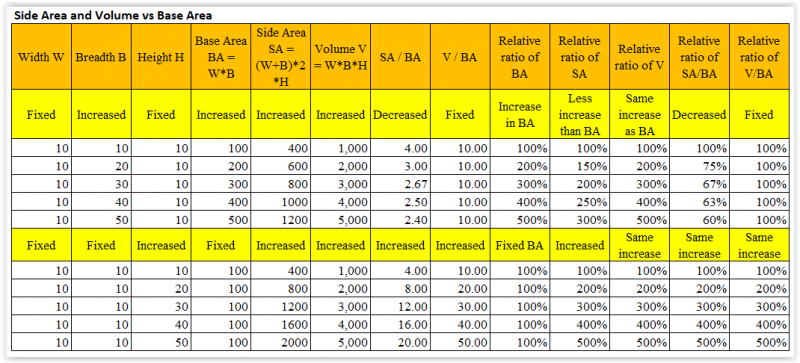​​​​​​
2. For the same height, the volume increases in proportion to the increase in the base area (i.e. fixed volume/base area), while the side area increases less rapidly resulting in a decrease in the side area/base area ratio:
•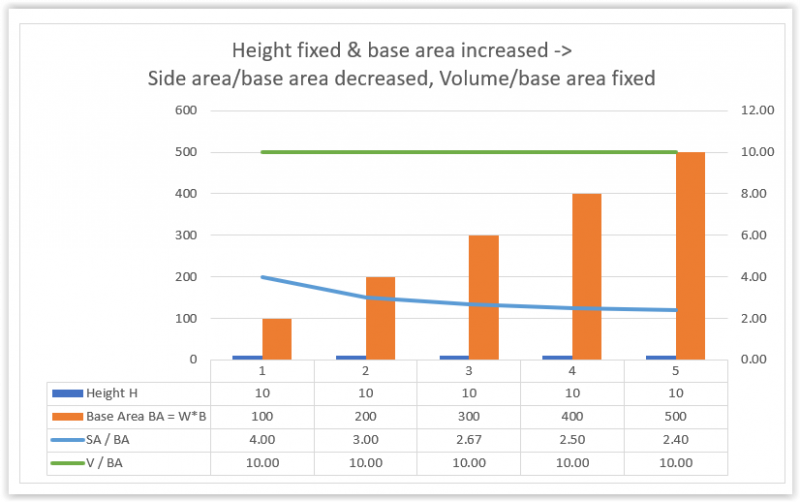3. For the same base area, the side area and volume increase in proportion to the increase in the height (as shown in the graph below). Their rates of change are the same:
•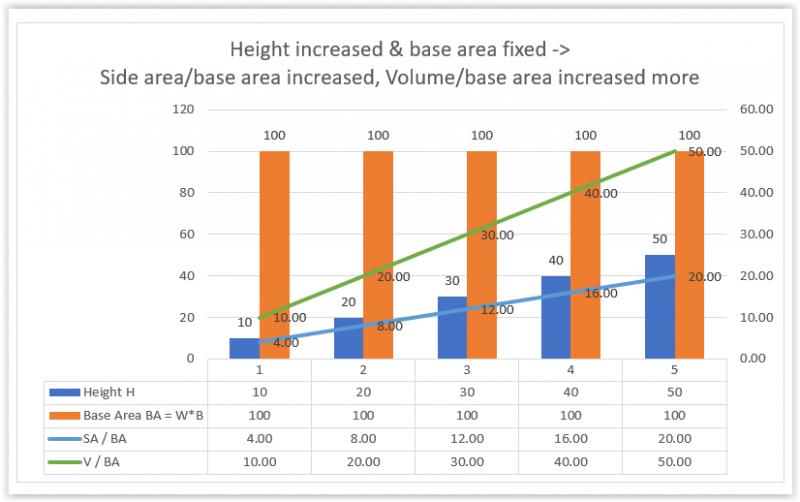4. If both the height and the base area are increased, the volume/base area will increase, but whether the side area/base area will increase or decrease will depend on the relative rates of changes of the height and the base area.
5. In all cases, the unit cost and unit sales value should therefore not remain constant.
6. The appropriate unit cost and unit sales value should be determined based on the quantity required, and suitably adjusted in case of changes in the quantity.

### Impact on unit cost and unit rate

1. Making a unit rate competitive enough to secure sales volume and produce profit is important.
2. Works or sales contracts usually require the unit rates to be fixed even though the quantities may be varied. If the variation is significant, there can be a huge loss or profit. Some contracts permit the adjustment of the unit rates in case of unexpected big changes.
3. The quantity of any item of work can vary between different construction projects and how to estimate an appropriate unit rate is crucial.

### Breakdown into more or less items

1. It would be more expedient if less items need to be measured to arrive at the total cost or price.
2. This means that the unit rate has to allow for the costs of other related items not measured separately.
3. However, when the costs of other related items do not vary in proportion to the quantity of the item measured, the unit cost of the item measured would change significant to become unrealistic, then such other related items should be measured separately for costing.
4. Therefore, the unit rates should only be required to include for related items which change in proportion to the quantity measured or for non cost significant ancillary items. Yet, significant changes to the quantity may still make the non cost significant ancillary items to become significant. This is an area for contractual claims.
-> Top

## 3. Building form and shape

1. Appreciate the effect of different site areas for the same building:​​​​​​​​​​​​
•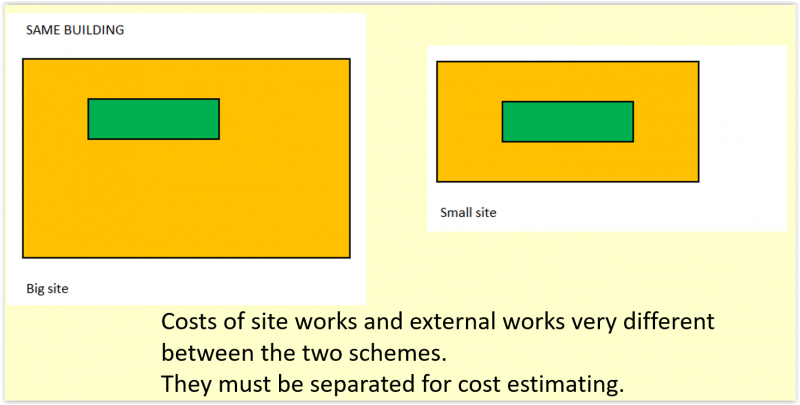2. Appreciate the effect of doubling the number of storeys:
•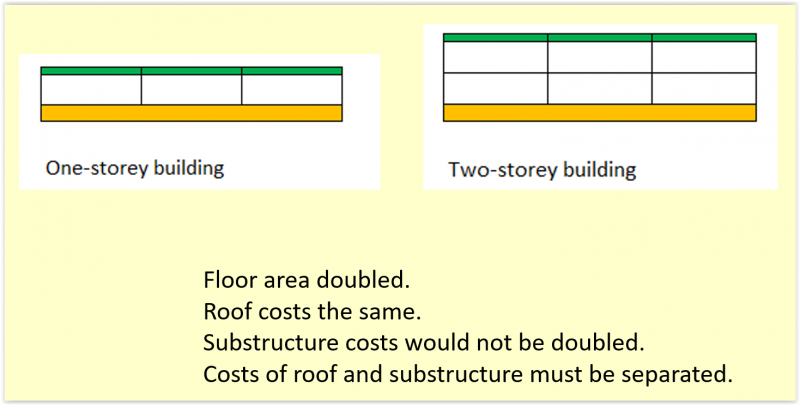3. Appreciate the effect of elongating the building without increasing the floor area:
•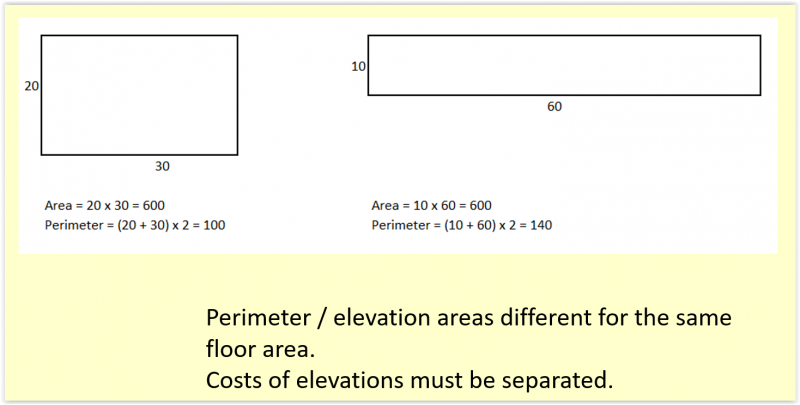4. ​​​​​​​Unit cost per floor area:
• Construction costs are usually compared based on unit cost per floor area.
• The above suggests that the unit cost per floor area cannot be a constant for the same type of buildings of different forms and shapes.
5. Impact on design:
• The relationship of number, length, area and volume is more important for the design of the building form and shape.
• Whether a building is more economical or expensive than the other for the same floor area and type would primarily depend on the ratios of the numbers, lengths, areas and volumes of the building components to the floor areas, and then on the materials and standards.​​​​​​​​​​​
-> Top

## 4. Most significant cost parameters

1.  Appreciate which cost portions should bear more direct proportions to each parameter:
​​​​​​​​​​​​​​​​​​​​​​​瞭解那部份的成本內容與那個造價參數有較直接的比例關係:​​​​​​​​​​​​​​
•​​​​​​​​​​​​​​
•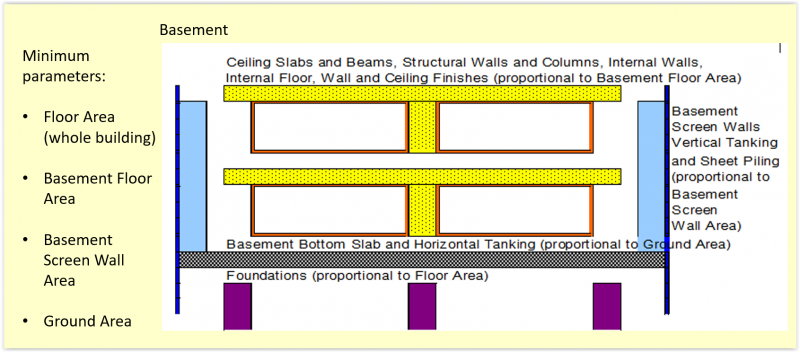2. Parameters:
• Floor area (above ground + below ground)
樓面面積
• Ground area (= roof area)
地面面積 （=屋面面積）
• Transfer structure area
轉換結構面積
• External elevation area
外立面面積
• Basement screen wall area or more effectively basement volume of excavation
地下室外牆面積或較有效的地下室土方體積
• Site area
工地面積
• External area (site area - ground area)
室外面積
• Number of equipment
台數
• Refrigeration tonnage
冷噸
• Other elemental quantities.
​​​​​​​其他功能分部工程量。
3. Quick cost estimating:
• Using this small number of cost parameters can enable quick cost estimating.
4. Accurate measurement:
• The values (quantities) of those parameters which will be used extensively throughout a cost estimate should be accurately measured, unlike the general message here that the cost estimates should be approximate.

End of Page

-> Top